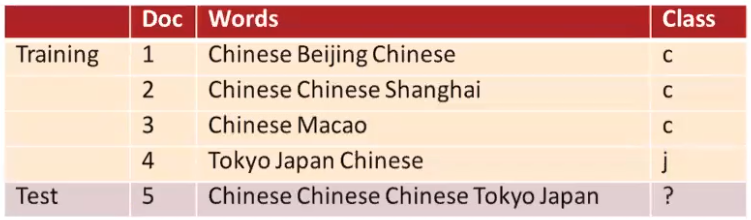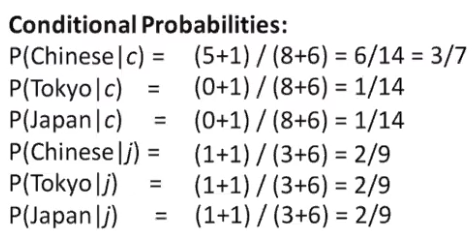std::bodun::blog

# Maximum Likelihood for Classification

Let’s say we want to classify an input text $$y$$ and give it a label $$x$$. Formally, we want to find:

$\textrm{argmax} P(x | y)$

By Bayes’ rule this is the same as

$\textrm{argmax} \frac{P(y|x)P(y)}{P(x)}$

Suppose we have five documents as training data and one document as the input as testing data. Our objective is to give a label to the test sentence.Credit: Eunsol Choi

Let’s define the probability of class as ($$N$$ is the total number of classes)

$p(x) = \frac{count(x)}{N}$

and the probability of a word appearing given a class label (total number of vocabs)

$p(w_i|x) = \frac{count(w_i,x) + 1}{count(x) + |V|}$

The conditional probabilities for $$p(w_i|y)$$ isNow, we want to find out which language label should we assign the sentence “Chinese Chinese Chinese Tokyo Japan”. This is the same as asking which labels ($$x$$)) should we pick so that $$P(W|x)P(x)$$ yields the greatest value. Mathematically, we want to find out where the gradient of the function $$P(W|x)P(x)$$ is flat.

If we label the sentence as j (Japanese), we have $$P(j | d_5) \propto \frac{1}{4}\cdot (\frac{2}{9}^3)\cdot \frac{2}{9}\cdot \frac{2}{9} \approx 0.0001$$. If we calculate $$P(c|d_5)$$, we get 0.0003, which generates the largest value for $$P(x | y)$$.